# Bihar Board Class 12th Physics Solutions Chapter 6 Electromagnetic Induction

Bihar Board Class 12 Physics Solutions Chapter 6 Electromagnetic Induction Textbook Questions and Answers, Additional Important Questions, Notes.

## BSEB Bihar Board Class 12 Physics Solutions Chapter 6 Electromagnetic Induction

### Bihar Board Class 12 Physics Electromagnetic Induction Textbook Questions and Answers

Question 1.
Predict the direction of induced current in the situations described by the following Figs, (a) to (f).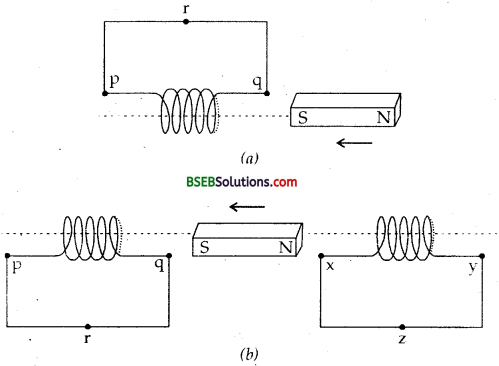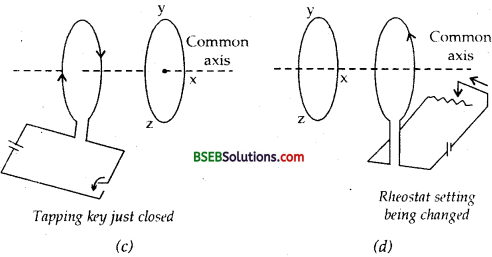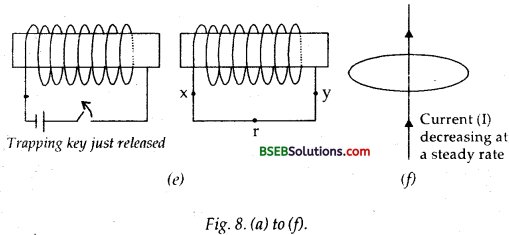(a) As the magnet moves towards the solenoid, the magnetic flux linked with the solenoid increases. According to Lenz’s law, the induced e.m.f. produced in the solenoid is such that it opposes the very cause producing it i.e., it opposes the motion of the magnet. Hence the face q of it becomes the south pole and p becomes north pole. Therefore, the current will flow along pq in the coil i.e., along q r pq in this figure i.e., clockwise when seen from the side of the magnet according to clock rule.

(b) As the north pole moves away from xy coil, so the magnetic flux linked with this coil decreases. Thus according to Lenz’s law, the induced e.m.f. produced in the coil will oppose the motion of the magnet. Hence the face. X becomes S-pole, so the current will flow in the clockwise direction i.e., along yzx in the cone. For coil pq, the sou th pole of the magnet mov es towards end q and thus this end will acquire south polarity so as to oppose the motion of the magnet, hence the current will flow along prq in the coil.

(c) The induced current will be in the anticlockwise direction, i.e., along yzx.
(d) The induced current will be in the clockwise direction i.e., along zyx.
(e) The battery current in the left coil will be from right to left, so by mutual induction, the induced current in the right coil will be in the opposite direction i.e., from left to right or along xry.
(f) In this case, there is no change in magnetic flux linked with the wire, so no current will flow through the wire since there is no induced current as the field lines lie in the plane of the loop.

Question 2.
Use Lenz’s law to determine the direction of induced current in the situation described by Fig. given below:
(a) A wire of irregular shape turning into a circular shape;
(b) A circular loop being deformed into a narrow straight wire.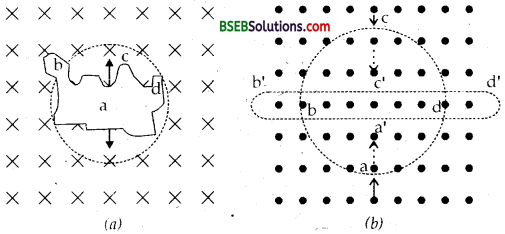(a) When a wire of irregular shape turns into a circular loop, the magnetic flux linked with the loop increases due to increase in area. The circular loop has greater area than the loop of irregular shape. The induced e.m.f. will cause current to flow in such a direction so that the wire forming the loop is pulled inward from all sides i.e., current must flow in the direction adcl^a as shown in Fig. (a) i.e., in anticlock-wise direction so that the magnetic field produced by the current (directed out of the paper) opposes the applied field.

(b) In Fig. (b), a circular loop deforms into a narrow straight wire i.e., upper side of loop should move downwards and lower end should move upwards to oppose the motion of the circular loop, thus its area decreases as a result of which the magnetic flux linked with it decreases. To oppose the decrease in magnetic flux, the induced current should flow anti clockwise in the loop, i.e., along a’d’c’b’a’. Due to the flow of anti-clockwise current, the magnetic field produced will be out of the
page and hence the applied field is supplemented.Question 3.
A long solenoid with 15 turns per cm has a small loop of area 2.0 cm2 placed inside the solenoid normal to its axis. If the current carried by the solenoid changes steadily from 2.0 A to 4.0 A in 0.1 s, what is the induced emf in the loop while the current is changing?
Here, n = number of turns per unit length of the solenoid = 15 turns cm-1
= 1500 turns m-1.
A = area of loop placed insider the solenoid = 2.0 cm2 = 2 x 10-4 m2.
I1 = 2.0 A, I2 = 4.0 A,
dl = I2 – I1 = 4 – 2 = 2A
dt = 0.1 s, $$\frac {dI}{dt}$$ = $$\frac {2}{0.1}$$ = 20 As-1,
e = induced e.m.f. in the loop = ?
We know that the magnetic field produced inside the solenoid is given by :
B = µ0 nl
lf ø be the magnetic flux linked with the ioop, then
ø = BA = µ0nlA.
∴ Using the relation, e = – $$\frac {dø}{dt}$$ we get
e = –$$\frac {d}{dt}$$(ø) = –$$\frac {d}{dt}$$(m0 nlA) = -µ0nA$$\frac {dl}{dt}$$
∴ magnitude of e is given by
e = µ0nA.$$\frac {dl}{dt}$$
= 4n x 10-7 x 1500 x 2 x 10-4 x 20
= 7.54 x 10-6 V = 7.5 x 10-6V.

Question 4.
A rectangular wire loop of sides 8 cm and 2 cm with a small cut is moving out of a region of uniform magnetic field of magnitude 0.3T directed normal to the loop. What is the emf developed across the cut if the velocity of the loop is 1 cm s-1 in a direction normal to the (i) longer side, (ii) shorter side of the loop? For how long does the induced voltage last in each case?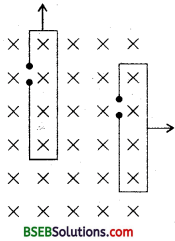Here, l = length of loop = 8 cm, b = breadth of loop = 2 cm
B = magnetic field = 0.3 T
v = Velocity of the loop = 1 cms-1 = 102ms-1
A = Area of loop = l x b = 8 x 2 x 10-4 m2 = 16 x 10-4 m2.
e = induced e.m.f. = ?
t = time for which e.m.f. lasts in the loop = ?

(i) When the velocity is normal to the longer side :
Using the relation, e = B/v, we get
e = 0.3 x 8 x 10-2 x 10-2 = 2.4 x 10-4 V = 0.24 x 10-3 V = 0.24 mV.

The e.m.f. will last in the loop till it does not get out of the magnetic field i.e., for the time the loop takes to travel a distance equal to the length of the shorter arm.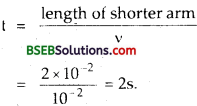(ii) When the velocity is normal to the shorter arm :
Here, b = 2 x 10-2 m,
e = Bbυ gives
e = Bbυ = 0.3 x 2 x 102 x 102 = 0.6 x 10-4 V = 0.06 x 10-3 = 0.06 mVQuestion 5.
A 1.0 m long metallic rod is rotated with an angular frequency of 400 rad s-1 about an axis normal to the rod passing through its one end. The other end of the rod is in contact with a circular metallic ring. A constant and uniform magnetic field of 0.5 T parallel to the axis exists everywhere. Calculate the emf developed between the centre and the ring.
Here, l = length of the metallic rod = 1.0 m.
ω = angular frequency = 400 rads-1.
B = Uniform magnetic field = 0.5 T
υ1 = Velocity of one end = 0.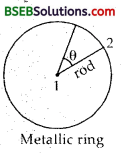Let e = induced e.m.f. between the centre and the ring = ?
υ2= Velocity of 2nd end = ωl (∵ υ = rω, here r = l)
If υ be the average velocity, then
υ = $$\frac{v_{1}+v_{2}}{2}=\frac{0+\omega l}{2}=\frac{\omega l}{2}$$
e = induced e.m.f. between the centre and the ring = ?
Using the relation, e = Bvl, we get
e = $$\frac{\mathrm{B} \omega l .1}{2}$$ = $$\frac{1}{2}$$ Bω l2
= $$\frac{1}{2}$$ x 0.5 x 400 x (1)2
= 100 VQuestion 6.
A circular coil of radius 8.0 cm and 20 turns is rotated about its vertical diameter with an angular speed of 50 rad in a uniform horizontal magnetic field of magnitude 3.0 x 10-2 T. Obtain ,the maximum and average emf induced in the coil. If the coil forms a closed loop of resistance 100, calculate the maximum value of current in the coil. Calculate the average power loss due to Joule heating. Where does this power come from?
Here, n = number of turns in the coil = 20
r = radius of coil = 8.0 cm = 8 x 10-2 m
ω = angular speed of the coil = 50 rad s-1
B = magnetic field = 3.0 x 10-2 T.
Let e0 be the maximum e.m.f. in the coil = ?
and eav be the average e.-m.f. in the coil = ?
We know that the instaneous e.m.f. produced in a coil is given by :
e = BA nW sin ωt.
for e to be maximum emax., sin ωt = 1.
emax = BAnω = B. πr2

where A = πr2 is the area of the coil :eav for one cycle is given by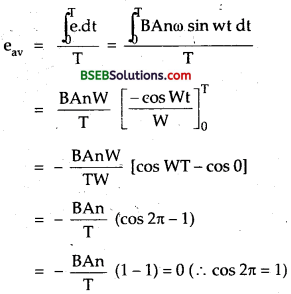i.e., eav is zero as the average value of sin wt for one complete cycle is always zero.
Now R = resistañof the closed loop formed by the coil = 10
Let Imax = max maximum current in the coil = ?
∴ Using the relation,

∴ eav for one cycle is given by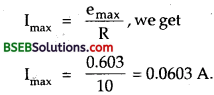Let Pav be the average power loss due to Joule heating =?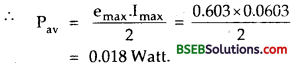The induced current causes a torque opposing the rotation of the coil. An external agent must supply torque and do work to counter this torque in order to keep the coil rotating uniformly. Thus the source of the power dissipated as heat in the coil is the external agent i.e., rotor.

Question 7.
A horizontal straight wire 10 m long extending from east to west is falling with a speed of 5.0 m s-1, at right angles to the horizontal component of the earth’s magnetic field, 0.30 x 10-4 Wb m2.
(a) What is the instantaneous value of the emf induced in the wire?
(b) What is the direction of the emf?
(c) which end of the wire is at the higher electrical potential?
Here, l = length of wire = 10 m.
υ = speed = 5.0 ms-1.
BH = horizontal component of earth’s magnetic field = 0.30 x 10-4 Wb m-2.

(a) Let e be the value of instantaneous induced e.m.f. in the wire.
∴ Using the relation,
e = Blυ,we get
e = BH
= 0.3 x 10-4 x 10 x 5
= 1.5 x 10-3 V.
(b) The induced e.m.f. will act in a direction so as to oppose the motion of the falling straight wire i.e., from west to east.
(c) As the induced e.m.f. opposes its cause i.e. it sets from low to
high potential end, thus eastern end is at higher potential as induced e.m.f. acts from West to East.Question 8.
Current in a circuit falls from 5.0 A to 0.0 A in 0.1 s. If an average emf of 200 V induced, give an estimate of the self-inductance of the circuit.
Here, I1 = initial current in the circuit = 5.0 A
I2 = Final current in the circuit = 0.0 A
∴ Change in current, dl = I2 – I1 = 0 – 5 = – 5 A
dt = time in which the current changes = 0.1 s
e = average induced e.m.f. = 200 V
L = Self inductance of the circuit = ?
Using the relation,
e = -L $$\frac{dl}{dt}$$
we get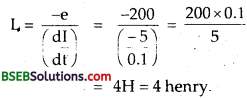Question 9.
A pair of adjacent coils has a mutual inductance of 1.5 H. If the current in one coil changes from 0 to 20 A in 0.5 s, what is the change of flux linkage with the other coil?
Here, M = Mutual inductance of two coil = 1.5 H
dl = change in current in one coil = I2 – I1 = 20 – 0 = 20 A.
dt = time in which the change in current takes place = 0.5 s.
dø = change in magnetic flux of the other coil = ?
If e be the induced e.m.f. produced in the other coil, then
Using the relation,
e = -M $$\frac{dI}{dt}$$, we get
e = – 1.5 x $$\frac{20}{0.5}$$ = – 60 V
Also we know that
e = – $$\frac{dø}{dt}$$
dø = -e x dt = -(-60) x 0.5 = 30 Wb.

Question 10.
A jet plane is travelling towards west at a speed of 1800 km/h. What is the voltage difference developed between the ends of the wing having a span of 25 m, if the Earth’s magnetic field at the location has a magnitude of 5 x 10-4 T and the dip angle is 30°.
Here, $$\overrightarrow{\mathrm{v}}$$ = 1800 km/h = 1800 x $$\frac {5}{18}$$= 500 ms-1 towards west.
l = wingspan = 25 m, B = earth’s magnetic field = 5 x 10-4T.
δ = angle of dip = 30°
.’. Bv = Vertical component of earth’s magnetic field
= B sin δ = 5 x 10-4 x sin 30 = 5 x 10-4 x $$\frac {1}{2}$$ = 2.5 x 10-4 T.
Bv is normal to both the wings and the direction of motion. So if e be the induced e.m.f. produced (= voltage difference developed between the ends of the wing), then
e = Bvl = 2.5 x 10-4 x 25 x 500 = 3125 x 10-3 V.
= 3.125 V = 3.1 V (Using significant figures).
The direction of the wing is immaterial as long as it is horizontal for this answerQuestion 11.
Suppose the loop in Exercise 6.4 is stationary but the current feeding the electromagnet that produces the magnetic field is gradually reduced so that the field decreases from its initial value of 0.3 T at the rate of 0.02 T s-1. If the cut is joined and the loop has a resistance of 1.6 Ω, how much power is dissipated by the loop as heat? What is the source of this power?
Here, A = 8 x 2 cm2 = 16 x 104 m2.
B1 = initial value of magnetic field = 0.3 T
$$\frac {dB}{dt}$$ = rate of decrease of the magnetic field = 0.02 Ts-1.
R = resistance of the loop = 1.6 Ω
P = power dissipated as heat = ?
‘ If 6 be the flux linked with the loop, then ø = BA
Thus the induced e.m.f. ‘e’ produced in the loop is given by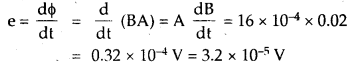the induced current I is given by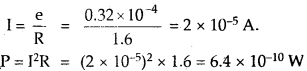The source of this power is the external agency changing the magnetic field with time.

Question 12.
A square loop of side 12 cm with its sides parallel to X and Y axes is moved with a velocity of 8 cm s’1 in the positive x-direction in an environment containing a magnetic field in the positive z-direction. The field is neither uniform in space nor constant in time. It has a gradient of 10-3 T cm-1 along the negative x-directipn (that is it increases by 10-3 T cm-1 as one moves in the negative x-direction), and it is decreasing in time at the rate of 10″3 T s-1. Determine the direction and magnitude of the induced current in the loop if its resistance is 4.50 mΩ.
Here, a = side of the square loop = 12 cm = 12 x 10-2 m.$$\overrightarrow{\mathrm{v}}$$ = Velocity of loop parallel to x-axis = 8 cms-1 = 8 x 10-2 ms-1
Let B = variable magnetic field acting away from us ⊥ ar to the XY plane along z axis i.e., plane of paper represented by x.
$$\frac {dB}{dX}$$ 10-3 T cm-1 = 10-3 x 102 T m-1 = o.l T m-1 = field gradient along -υe x direction.
$$\frac {dB}{dX}$$ = rate of variation with time
= 10-3 Ts-1
R = resistance of the loop = 4.50 mΩ = 4.5 x 103 Ω.
Let I = induced current = ? and its direction = ?
∴ A = area of loop = a2 = (12 x 10-2)2 m2 = 144 x 10-4m2.
The magnetic flux changes (i) due to the variation of B with time
and (ii) due to motion of the loop in non-uniform $$\overrightarrow{\mathrm{B}}$$ .
Thus if ø be the total magnetic flux of the loop, then ø is calculated as :

Let dø = magnetic flux linked with shaded part = B (x, t) a dx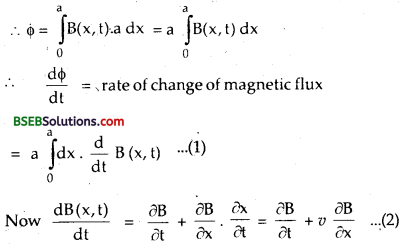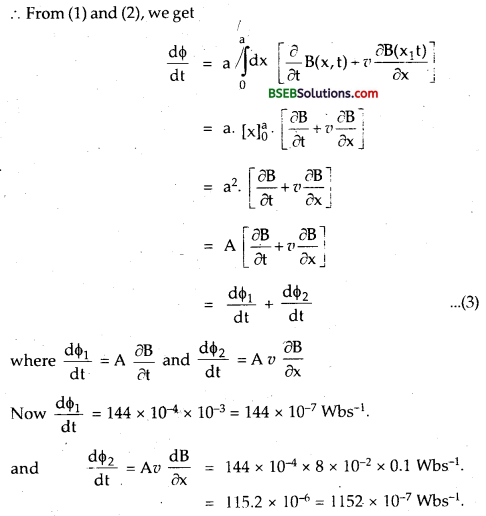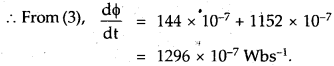Clearly the two effect add up as these cause a decrease in flux along the + z direction.
∴If e be the induced e.m.f. produced, thenThe direction of induced current is such as to increase the flux through the loop along + z-direction. Thus if for the observer, the loop moves to the right, the current will be seen to be anti-clockwise.

Question 13.
It is desired to measure the magnitude of field between the poles of a powerful loud speaker magnet. A small flat search coil of area 2 cm2 with 25 closely wound turns, is positioned normal to the field direction, and then quickly snatched out of the field region. Equivalently, one can give it a quick 90° turn to bring its plane parallel to the field direction). The total charge flown in the coil (measured by a ballistic galvanometer connected to coil) is 7.5 mC. The combined resistance of the coil and the galvanometer is 0.50 Q. Estimate the field strength of magnet.
Here, A = area of coil = 2 cm2 = 2 x 104 m2.
N = number of turns = 2 s.
q = charge flown in the coil = 7.5 mC = 7.5 x 10-3 C
R = resistance of coil and galvanometer = 0.50 Ω.
Let B = field strength of the magnet = ?
Let ø1 = magnetic flux linked with the search coil initially :
.’. ø1 = NBA.
Also let 2 = magnetic flux linked with the coil finally when it is brought out of the field.
ø2 = 0
If e be the induced e.m.f. produced in the coil, then
e = – $$\frac {dø}{dt}$$
According to Ohm’s Law, e = IR, where I = induced current = $$\frac {dq}{dt}$$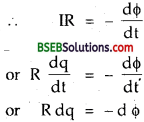integrating both sides of (i), we get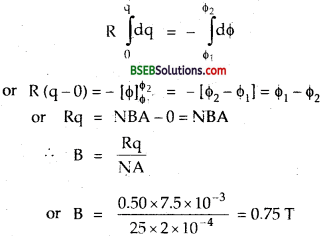Question 14.
Figure shows a metal rod PQ resting on the smooth rails AB and positioned between the poles of a permanent magnet. The rails, the rod, and the magnetic field are in three mutual perpendicular directions. A galvanometer G connects the rails through a switch K. Length of the rod = 15 cm, B = 0.50 T, resistance of the closed loop containing the rod = 9.0 mO. Assume the field to be uniform.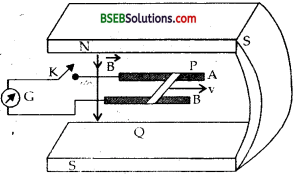(a) Suppose K is open and the rod is moved with a speed of 12 cm s-1 in the direction shown. Give the polarity and magnitude of the induced emf.
(b) Is there an excess charge built up at the ends of the rods when K is open? What if K is closed?
(c) With K open and the rod moving uniformly, there is no net force on the electrons in the rod PQ even though they do experience magnetic force due to the motion of the rod. Explain.
(d) What is the retarding force on the rod when K is closed?
(e) How much power is required (by an external agent) to keep the rod moving at the same speed (=12 cm s-1) when K is closed? How much power is required when K is open?
(f) How much power is dissipated as heat in the closed circuit? What is the source of this power?
(g) What is the induced emf in the moving rod if the magnetic field is parallel to the rails instead of being perpendicular?
Here, B = 0.50 T
l = length of the rod = 15 cm = 15 x 10-2 m.
R = resistance of the closed loop containing the rod = 9.0 mΩ = 9 x 10-3Ω.
(a) v = speed of the rod = 12 cms-1 = 12 x 10-2 ms-1.
The magnitude of the induced e.m.f. is
E = Blυ = 0.50 x 15 x 10-2 x 12 x 10-12
= 9 x 10-3V
According to Fleming’s left hand rule, the direction of Lorentz
force $$\overrightarrow{\mathrm{F}}$$ = -e( $$\overrightarrow{\mathrm{V}}$$ x $$\overrightarrow{\mathrm{B}}$$ on electrons in PQ is from P to Q. So the end P of the rod will acquire positive charge and Q will acquire negative charge.

(b) Yes. When the switch K is open, the electrons collect at the end Q, so excess charge is built up at the end Q. But when the switch K is closed, the accumulated charge at the end Q is maintained by the continuous flow of current.

(c) This is because the presence of excess charge at the ends P and Q of the rod sets up an electric field E . The force due to the electric field
(q $$\overrightarrow{\mathrm{E}}$$) balances the Lorentz magnetic force q ($$\overrightarrow{\mathrm{v}}$$ x $$\overrightarrow{\mathrm{B}}$$ ). Hence the net force on the electrons is zero.

(d) When the key K is closed, current flows through the rod. The retarding force experienced by the rod is:
F=BIl = B($$\frac{E}{R}$$)
Where I = $$\frac{E}{R}$$ is the induced current.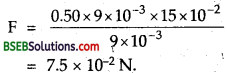(e) The power required by the external agent against the above retarding force to keep the rod moving uniformly at speed 12 ems-1 when ‘ K is dosed is given by ,
P = FV = 7.5 x 10-2 x 12 x 10-2
= 90 x 10-3 W = 9 x 10-3 W.

(f) Power dissipated as heat is given by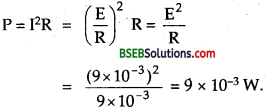The source of this power is the power provided by the external agent calculated in (e)

(g) Zero. This is because when the magnetic field is parallel to therails, θ = 0°, so induced e.m.f. E = Blυ sin θ = 81v sin 0 = 0. In this situation, the moving rod does not cut the field lines, so there is no change in the magnetic flux, hence E = 0.Question 15.
An air-cored solenoid with length 30 cm, area of cross – section 25 cm2 and number of turns 500, carries a current of 2.5 A. The current is suddenly switched off in a brief time of 10-3 s. How much is the averageback emf induced across the ends of the open switch in the circuit? Ignore the variation in magnetic field near the ends of the solenoid.
Here, l = length of the solenoid = 30cm = 30 x 10-2m.
A = area of cross-section = 25 cm2 = 25 x 10-4 m2.
N = total no. of turns = 500
I1 = current in the solenoid before switching = 2.5 A
I2 = current in the solenoid after switching = 0
dt = time of change of current 10-3 s.
Let E average back e.m.f. induced in the solenoid.
If n be the no. of turns per unit length, then
n = $$\frac{\mathrm{N}}{\mathrm{l}}=\frac{500}{30 \times 10^{-2}}=\frac{5000}{3}$$
We know that the magnetic field inside the solenoid is given by
B = µ0nI = 4π x 10-7 x $$\frac{5000}{3}$$. x 2.5 = 52.36 x 10-4T.
If ø1 be the initial magnetic flux linked with the solenoid,
Then ø1 = NBA = 5000 x 52.36 x 10-4 x 25 x 10-4 = 65.45 x 10-4 Wb.
ø2 = final flux = 0 as I2 = 0.

∴ dø = change in magnitude flux = ø2 – ø1
= – 65.45 x 10-4 Wb.
∴ E = $$\frac{dø}{dt}$$ = $$\frac{65.45 \times 10^{-4}}{10^{-3}}$$ = 6.545 V.

Question 16.
(a) Obtain an expression for the mutual inductance between a long straight wire and a square loop of side a as shown in Fig. here.
(b) Now assume that the straight wire carries a current of 50 A and the loop is moved to the right with a constant velocity, v = 10 m/s. Calculate the induced emf in the loop at the instant when x = 0.2 m. Take ∝ = 0.1 m and assume that the loop has a large resistance.(a) Here, the side of the square loop = a
Current in the long straight wire = I
The straight current carrying wire produces magnetic field around it.
Hence a magnetic flux is linked with the square ioop moving with a velocity υ ms-1.
Let B be the magnetic field produced by the long straight wire at a distances r from it, then
B = $$\frac{\mu_{0}}{4 \pi} \cdot \frac{2 \mathrm{I}}{\mathrm{r}}$$ ……(1)
and it acts normal to the plane of the loop.
Let the square ioop be divided into a large number of elementary strips. Consider one such styip of width dr at a distance r from the wire.
If dA be the area of this strip, then
If dø be the magnetic ílpx linked with the strip, then dø = BdA =
$$\frac{\mu_{0}}{4 \pi} \cdot \frac{2 \mathrm{I}}{\mathrm{r}}$$ = $$\frac{\mu_{0}}{2 \pi} \cdot \frac{2 \mathrm{I}}{\mathrm{r}}$$ ……(2)
If ø be the total magnetic flux linked with the square loop, thenNow let M be the mutual inductance between the straight wire and the loop.
ø = MI ………..(4)
From (3) and (4), we get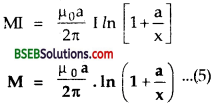Which is the required expression for M.
(b) Here, x = 0.2 m, a = 0.1 m,
V = 10 ms-1,
I= 50 A.
Let e = induced e.m.f. in the loop = ?
We know thatPutting values of I, a, υ, x, we get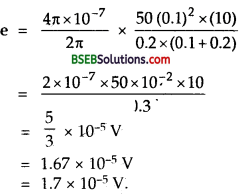Question 17.
A line charge X per unit length is lodged uniformly onto the rim of a wheel of mass M and radius R. The wheel has light non¬conducting spokes and is free to rotate without friction about its axis as shown in fig here. A uniform magnetic field extends over a circular region within the rim. It is given by,
B = -B0k (r<a;a<R)
= 0 (otherwise)
What is the angular velocity of the wheel after the field is suddenly switched off?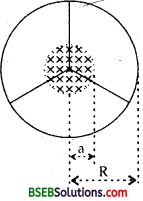Let ω be the angular velocity of the wheel of mass M and radius R.
Let e = Induced e.m.f. produced.
The rotational K.E. of the rotating wheel = $$\frac {1}{2}$$ Iω2 ……….(1)
where I = Moment of inertia of wheel
= $$\frac {1}{2}$$ MR2 …………(2)

Also by clef. ole.m.f.,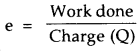or Work done = eQ
Applying the work energy theorem, we get
Rotational K.E. = Work done
or Rotational K.E. = Q x e …..(3)
We know that the e.m.f. of a rod rotating in a uniform magnetic
field is given by $$\frac {1}{2}$$ Bωa2, since here the magnetic field is changing, we assume the average over the time span and thus average value of e.m.f. is given by.’. Using equation (1) to (5), we getAliter:
When the $$\overrightarrow{\mathrm{B}}$$ is suddenly brought to zero, change in magnetic field occurs and is given by $$\frac{\mathrm{d} \overrightarrow{\mathrm{B}}}{\mathrm{dt}}=-\frac{\mathrm{d}}{\mathrm{dt}}\left(\mathrm{B}_{0} \hat{\mathrm{k}}\right)$$ ………..(1)Due to change in magnetic field, an electric field E is produced
which exerts a force qE on a mobile charge q. The work done in moving
the charge once around the ioop is given by
W = Force distance
= qE.2πR
if e = induced e.m.f., then
e = $$\frac {W}{q}$$ = $$\frac {qE}{q}$$.2πR=E.2πR ………(3)
Also we know that∴ From (3) and (4), we get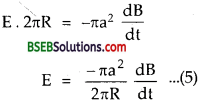Also we know that impulse due to electric field is given by
J = F. dt = qE dt = change in momentum of wheel
or qE dt = mv – 0 = m. Rw
or E = $$\frac {mRω}{qdt}$$ ……….(6)

where ω = angular speed of wheel
∴ From (5) and (6), we get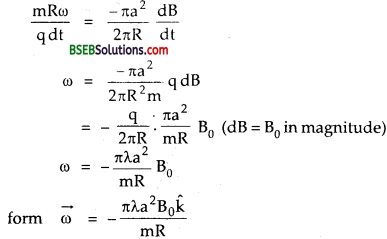Hence, proved.

### Bihar Board Class 12 Physics Chapter 6 Electromagnetic Induction Additional Important Questions and Answers

Question 1.
Define electromagnetic induction (E.M.I.).
It is defined as the process of generating induced e.m.f. or induced current in a circuit by changing the magnetic flux linked with it.

Question 2.
Why E.M.I. is an important phenomenon?
E.M.I is an important phenomenon because it is the basis of working of dynamos, power generators, transformers etc.

Question 3.
Define magnetic flux through a surface. How is it measured? Explain.
It is defined as the total number of magnetic lines of force passing through the given surface normally. It is measured as the product of the component of magnetic field normal to the surface and the surface area. It is denoted by ø .
∴ Let θ = angle made by $$\overrightarrow{\mathrm{B}}$$ with the normal to the area element $$\overrightarrow{\mathrm{dS}}$$ .
∴Bn = Component of magnetic field normal to $$\overrightarrow{\mathrm{ds}}$$ = B cos 0.
∴ By def. if dø be the flux through $$\overrightarrow{\mathrm{ds}}$$ ,
Then dø = Bn ds = B cos θ ds
= B ds cos θ
= B . ds
.’. If ø be the total flux through the whole surface S, then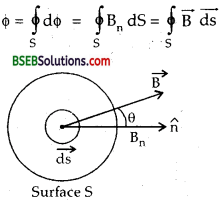Question 4.
Define induced e.m.f. What is the basic cause of induced
It is defined as the e.m.f. produced in a circuit by changing the current flowing through it or by changing the magnetic flux linked with it. .
The basic cause of induced e.m.f. is the change in magnitude flux.

Question 5.
State Lenz’s law.
It states that the induced current or induced e.m.f. produced in a circuit always flows in such a direction that it opposes the very ’cause or change which produces it.Question 6.
State Fleming’s Right hand Rule.
It states that if we stretch the first finger, central finger and the thumb of our right hand mutually perpendicular to each other such that the first finger points in the direction of the magnetic field and the thumb points in the direction motion of the conductor then, the central finger would point in the direction of the induced e.m.f. or current.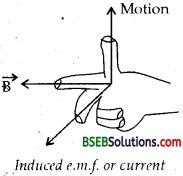Question 7.
What are various methods by which the induced e.m.f. in a circuit can be produced?
The following are the various methods of producing induced e.m.f. in a circuit:
(i) By changing the area of the circuit.
(ii) By changing the magnitude of the magnetic field ( B ).
(iii) By changing the relative orientation of the coil w.r.t. the
magnetic field i.e., by changing the angle 0 between B and normal to the plane of coil.

Question 8.
Does charge in magnetic flux induce e.m.f. or current?
The e.m.f. is always induced in a circuit whenever there is a change of magnetic flux linked with it, but the current will be induced only when the circuit is complete.

Question 9.
Define motional e.m.f.
It is defined as the induced e.m.f. produced in a closed circuit or a wire by changing its area on moving it through a steady magnetic field.

Question 10.
Define eddy currents.
They are defined as the currents induced in*the form of closed loops in the body of a conductor due to the change in the magnetic flux linked with it when placed inside a magnetic field. They are also called Foucault currents.

Question 11.
Define self-induction.
It is defined as the property of a coil due to which it opposes any change in current or the magnetic flux linked with it by producing an induced e.m.f. in the coil itself.

Question 12.
Define coefficient of self induction of a coil.
It is defined to be numerically equal to the amount of magnetic flux linked with the coil when unit current flows through it.
Or
It is defined to be numerically equal to the e.m.f. induced in the coil when the rate of change of current through the coil is unity.

Question 13.
Define mutual induction.
It is defined as the process of producing induced e.m.f. in a coil by changing the current or magnetic flux linked ^with the neighbouring coil.

Question 14.
Define coefficient of mutual induction of two coils.
It is defined to be numerically equal to the amount of magnetic flux linked with one coil when unit current flows through the neighbouring coil
It is defined to be numerically equal to the e.m.f. induced in one coil when the rate of change of current is unity through the neighbouring coil.Question 15.
What factors govern the magnitude of the e.m.f. induced in an electric circuit?
The magnitude of the induced e.m.f. in an electric circuit is directly proportional to the rate of change of magnetic flux linked with the circuit.

Question 16.
Why the induced e.m.f. is also called back e.m.f. ?
It is called so because the induced e.m.f. always opposes the applied voltage.

Question 17.
What is the magnitude of the induced current in the circular loop KLMN of radius r in the figure given here, if the straight wire PQ carries a steady current of magnitude I ampere.No induced current will be produced in the circular loop. It is because, the magnetic flux linked with the loop does not change due to the steady current through the wire PQ.

Question 18.
Why the inductance coils are made of copper?
The inductance coils made of copper have very small ohmic resistance. Due to change in magnetic flux, a large induced current will be produced in such an inductance, and it offers appreciable opposition to the flow of current due to the applied e.m.f.

Question 19.
A coil is wound on an iron core and looped back on itself so that the core has two sets of closely wound wires in series carrying current in the opposite series. What do you expect about its self inductance? Will it be large or small?
The self-inductance will be small due to the cancellation of inductive effect. It is because, currents in two set of wires flow in the opposite directions and produce flux in opposite directions.

Question 20.
Why the coils in the resistance boxes are made from doubled up insulated wire?
It is done so as to cancel the effect of self-induced e.m.f. in the coil. The inductive effect in the two wires in a coil made of doubled up wire will be opposite to each other.

Question 21.
What are the dimensions of magnetic flux?
We know that the magnetic flux linked with a surface is given by:
ø = BA cos θ
= $$\frac {F}{qV}$$ A cos θ (∴ F = qVB)
∴ Dimensions of bare given by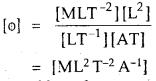Question 22.
Twelve wires of equal lengths are connected in the form of a cube which is moving with a velocity $$\overrightarrow{\mathrm{V}}$$ in the direction of $$\overrightarrow{\mathrm{B}}$$ .Find the induced e.m.f. in each arm of the cube.
We know that the force on a charged particle moving in a magnetic field is given by
$$\overrightarrow{\mathrm{F}}$$ = q($$\overrightarrow{\mathrm{V}}$$ x $$\overrightarrow{\mathrm{B}}$$) .Here, $$\overrightarrow{\mathrm{V}}$$ x $$\overrightarrow{\mathrm{B}}$$ as θ = 0° between them; so the force on electrons will be zero in any arm of the cube. As such there cannot be drift of electrons in any arm from its one end to the other. Hence no induced e.m.f. will be produced in any arm of the cube. ¦

Question 23.
Explain why self-induction is called the inertia of electricity?
Self-induction of a coil is its property due to which the coil opposes any change in the current flowing through it. It is because, the induced e.m.f. produced opposes the change in current. Hence it is called inertia of electricity. –

Question 24.
Spark is produced in switch when the light is put off. Explain why?
When the light is put off, a large induced e.m.f. is produced w’hich opposes the decay of current in the circuit. Hence the large current flows momentarily which causes sparking in the switch.

Question 25.
Two identical loops, one of copper and other of aluminium are rotated with the same speed in the same magnetic field. In which case the induced (a) e.m.f. (b) current will be more? Explain.
(a) The change in magnetic flux linked with both the loops will be same. So the induced e.m.f. produced in both the loops is same.
(b) As I = $$\frac {e}{R=[]’/}$$ and the resistance of copper loop is less than that of
the aluminium loop, so more current will flow through the copper loop than that in the aluminium loop.Question 26.
A coin is dropped between the pole pieces of a strong magnet. It falls as if moving through a highly viscous field. Why?
When the coin is dropped, eddy currents are produced in it. These eddy currents oppose the motion of the coin. Hence it appears as if moving in a highly viscous field.

Question 27.
Two inductors L1 and L2 sufficient distant apart are connected (a) in series, (b) in parallel. What is their equivalent inductance.
(a) In series combination, Ls = L1 + L2
(b) In parallel combinationQuestion 28.
State whether the following statements are true or false giving a brief reason:
(i) The dimensions of and (hie) are same.
(ii) An e.m.f. can be induced between the two ends of a straight copper wire when it is moved through a magnetic field.
(iii) A coil of a metal wire is kept stationary in a non-uniform magnetic field. An e.m.f. is induced in the coil.
(i)True,as $$\frac {h}{e}$$ =$$\frac{J-s}{\text { Coulomb }}$$ = $$\frac{\text { Joule }}{\text { Ampere }}$$ = [ML2T2A-1]
e Coulomb Ampere
Also ø = [ML2T2A-1]
(ii) True, as e = Blυ sin θ.
(iii) False. As the magnetic Ilux does not change for the stationary coil.

Question 29.
Define one henry.
One henry is the self-inductance oía coil in which an induced ne volt is induced in the coil when the rate of change of current is one ampere/sec.

Question 30.
how eddy currents can be minimised?
The metal core to be used in an appliance like transformer, choke coil, dynamo etc. is taken in the form of thin sheets i.e’. each sheet is electrically insulated from the other by varnish. Such a core is called laminated core.

Question 31.
What are undesirable effects of eddy currents?
The resistance of a metallic conductor is quite low, the magnitude of eddy currents produed is quite large, so large amount of heat is produced in the conductor according to Joule’s law of heating effect of current. Thus il large eddy currents are allowed to produce in the core of a choke coil, transformer etc, it may produce undesirable effects.Question 32.
What is the sefl-inductance of a solenoid if the rate of change of current of 4 A s-1 induces in it an e.m.f. of 20 mV?
Here, e = 20 mV = 20 x 10-3V, $$\frac {dl}{dt}$$ = 4 As-1
e = L $$\frac {dl}{dt}$$ gives
L = $$\frac{\mathrm{e}}{\left(\frac{\mathrm{dI}}{\mathrm{dt}}\right)}$$ = $$\frac{20 \times 10^{-3}}{4}$$ = 5 x 10-3 H

Question 33.
Calculate the induced e.m.f. in a coil in which the magnetic flux threading the coil changes from 12 x 10-3 Wb to 6 x 10-3 Wb in 0.01 s.
Here, dø = ø2 – ø1 = 6 x 10-3 – 12 x 10-3 = – 6 x 10-3 Wb. dt= 0.01 s, e = ?
∴ e = – $$\frac {dø}{dt}$$ = $$\frac{6 \times 10^{-3}}{0.01}$$ = 0.6 V.

Question 34.
State the limitation of eddy currents.
(i) They oppose the relative motion.
(ii) They involve loss of energy in the form of heat.
(iii) The excessive heat produced may break the insulation in the device and thus reduces its life.

Question 35.
To what types of circuits is Lenz’s law applicable? Why?
Lenz’s law is applicable to closed circuits only because it refers to the induced currents.

Question 36.
Define an ideal inductor.
An electric circuit element like a tightly wound coil of wire having very high value of self inductance is called an inductor and the inductor having high value of self induction and zero ohmic resistance is called an ideal inductor.

Question 37.
State the principle on which a.c generator works.
It works on the principle of electromagnetic induction which states that when a’ coil is rotated in a uniform magnetic field, an induced e.m.f. is produced in it.

Question 1.
The following are the three laws of E.M.I. given by Faraday.
(i) An induced e.m.f. is always produced in a circuit or a coil whenever there is a change of magnetic flux linked with it.
(ii) The induced e.m.f. lasts so long as the change in the magnetic flux continues.
(iii) The magnitude of the induced e.m.f. is directly proportional to the rate of change of the magnetic flux linked with the circuit.
i.e., mathematically, e ∝ $$\frac{\phi_{2}-\phi_{1}}{\mathrm{t}}$$
or e = -k ($$\frac{\phi_{2}-\phi_{1}}{t}$$)
where k is proportionality constant.
ø1 ø2are initial and final values of magnetic flux linked with the coil.
t = time in which the flux changes from to ø1 to ø2 Negative sign shows that e is of opposing nature. In S.l. system, k = I
e = $$\frac{\phi_{2}-\phi_{1}}{t}$$
or e = – $$\frac {dø}{dt}$$
If the coil has N turns, and Ø be the flux of each turn, then
e = – $$\frac {d}{dt}$$ (Nø) = – N$$\frac {dø}{dt}$$ .Question 2.
Prove that Lenz’s law is in accordance with the law of conservation of energy.
In the electromagnetic induction, the electrical energy (in the form of induced current or induced e.m.f.) is produced at the cost of mechanical energy. To varify it, let us consider a closed coil connected to a galvanometer. Let a bar magnet faces with its N-pole towards coil. Now move the magnet towards the coil. The magnetic flux linked with it will go on increasing, thus induced e.m.f. is produced in the coil. Due to this, induced current flows through the galvanometer which shows the deflection. According to Lenz’s law, the direction of induced e.m.f. is such that it opposes the cause z.e., motion of magnet which has produced it. To oppose the cause, the upper face of the coil acquires N-polarity, thus repels the magnet. To move the magnet towards the coil, mechanical work has to be done to overcome the force of repulsion between the N-poles. This work done is converted into electrical energy.Similarly when the magnet moves away from the coil, it face near the magnet acquires S-polarity so as to oppose its motion. Again the work has to be done to overcome the force of attraction between S and N-pole of the coil and magnet respectively and the work is converted into electrical energy. Hence Lenz’s Law is in accordance with the law of conservation of energy.

Question 3.
Desire the expression for the motional e.m.f.
Consider a closed circuit ABCD. Let a uniform magnetic field
$$\overrightarrow{\mathrm{B}}$$ be directed into the plane of paper and this circuit is placed in $$\overrightarrow{\mathrm{B}}$$
Let $$\overrightarrow{\mathrm{V}}$$ be the velocity of the circuit towards right. If $$\overrightarrow{\mathrm{f}_{\mathrm{m}}}$$ be the magnetic Lorentz force experienced by each electron inside the wire, then
$$\overrightarrow{\mathrm{f}_{\mathrm{m}}}$$ = -e ($$\overrightarrow{\mathrm{V}}$$ x $$\overrightarrow{\mathrm{B}}$$) ……..(1)
where $$\overrightarrow{\mathrm{f}_{\mathrm{m}}}$$ = – e charge on an electron and acts along BC.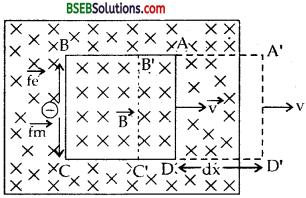Due to this force the electrons accumulate at end C, thus make C negatively charged while B is positively charged. Hence an electric field $$\overrightarrow{\mathrm{E}}$$ is set up between the two ends of the wire BC along B to C, so the electrons experience an electrostatic force $$\overrightarrow{\mathrm{f}_{\mathrm{e}}}$$ = – e $$\overrightarrow{\mathrm{E}}$$ along C to B under equilibrium condition, the net force on an electron becomes zero i.e.,If ε be the induced e.m.f. produced in arm BC of length l, then
E = $$\frac {ε}{l}$$ ………(4)
From (3) and (4), we get
$$\frac {ε}{l}$$ = υB
or ε = υlB which is the required expression for the motional e.m.f.Aliter :
Let dø be the decrease in the magnetic flux of the circuit ABCD when it moves out of magnetic field by a small distance dx in a time dt. ‘
dø = – B. x BC x dx = – B, l dx, where l = length
If ε be the induced e.m.f. produced in the circuit, then according to Faraday’s rule,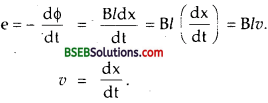If R be the resistance of the closed circuit, then the induced current flowing through the circuit is given by
I = $$\frac {E}{R}$$ = $$\frac {Blυ}{R}$$

Question 4.
Derive the expression for the force required to pull a conductor or a rod out of magnetic field.
Let υ be the velocity of the conductor of kngth I moving through a constant magnetic field $$\overrightarrow{\mathrm{B}}$$ .
Let I = Current flowing through the conductor.
If E be the motional e.m.f. produced in the conductor, then
I = $$\frac {ε}{R}$$ = $$\frac {Blυ}{R}$$ ……..(1)
Due to this current in the conductor, it experiences a force. To pull the conductor with a constant speed z’, this force must be balanced by the pulling force.
Let F be the pulling force.
∴ Fυ = mechanical power supplied to the circuit or conductor …(2)
Also electric power = rate at which electric energy is supplied = rate at which electric work s done.
= ε.l = $$\frac{\varepsilon^{2}}{\mathrm{R}}=\frac{(\mathrm{B} l v)^{2}}{\mathrm{R}}$$ ……..(3)
∴From (2) and (3), we get
Fυ = $$\frac{\mathrm{B}^{2} l^{2} v^{2}}{\mathrm{R}}$$
or F = $$\frac{\mathrm{B}^{2} l^{2} v}{\mathrm{R}}$$
which is the required expression for thL pulling force.

Question 5.
What are the advantages or application of eddy currents?
The following are the advantages of eddv currents.
(i) Diathermy : These are used for deep heat treatment i.e., localised heating of tissues in human body. This treatment is called Diathermy.
(ii) Concept of eddy currents is used in energy metres to record the consumption of electricity.
(iii) Speedometer : The eddy currents are produced in the aluminium drum in which a magnet attached to the axle of the wheel rotates according to the speed of the vehicle. The pointer attached to the drum is deflected in the direction of rotation of the drum.
(iv) Electromagnetic brakes : These are used in trains. The large eddy currents are produced in the metallic drum which oppose the motion of the drum.
(v) Dead beat galvanometer : Electromagnetic damping is used to stop coils in a shorter interval of time. When the coil wound over a metallic frame is deflected, eddy currents are produced ‘ in the metallic frame which oppose the motion of the coil.
(vi) Induction Furnace makes use of heating effect of edd v currents.
(vii) Induction motor or a.c. motor also works bv eddv currents.

Question 6.
Prove that e = – L $$\frac {dI}{dt}$$ where the symbols have their usual meanings.
Let I be the current flowing through a coil. Also let (j) be the magnetic flux linked with the coil at any instant. It is found that at any instant,
ø ∝ I.
or ø ∝ LI ……(i)
Where L is the proportionality constant known as coefficient of self-induction. If s be the induced e.m.f. produced in the coil, then
ε = – $$\frac {dø}{dt}$$ = –$$\frac {d}{dt}$$(LI) = -L$$\frac {dI}{dt}$$.

Question 7.
Prove that c or e =  M where the symbols have their usual meaning.
Let P and S be the two coils as shown in figure. If I be the current flowing through the P coil at any instant, then the magnetic flux linked with the S-coil at that instant is found to be directly proportional to I i.e.,
ø ∝ I
ø ∝ MI
where M is the coefficient of mutual induction or simply mutual- induCtance of two coils.If s be the induced e.m.f. produced in the S-coil, then
ε = – $$\frac {dø}{dt}$$ = –$$\frac {d}{dt}$$(MI) = –$$\frac {dI}{dt}$$.

Question 8.
On what factors does the coefficients of mutual inductance depends?
It depends upon the following factors:
(i) Total number of turns in the two coils.
(ii) Nature of the material on which the two coils are wound.
(iii) Size of the coils.
(iv) Distance between two coils.
(v) Relative placement of two coils i.e., their orientation.Question 9.
On what factors does the coefficient of self-inductance of a given coil depends?
It depends upon:
(i) The number of turns in the coil.
(ii) area of cross-section of the coil. More the area of the conductor, lesser will be its resistance and thus more is the rate of change current and thus lesser will be L.
(iii) the permeability of the medium over which the col is wound.
(iv) the length of the coil.

Question 10.
Derive the expression for self-inductance of a long-solenoid?
A solenoid is said to be long if its length is very large as compared to its radius of the cross-section.
Let N = total no. of turns in the solenoid of length l and area of cross-section A.
If n be the no. of turns per unit length of the solenoid, then
n = $$\frac {N}{l}$$ ……….(i)
Let I be the current flowing through the solenoid. If B be the magnetic field at a point inside the solenoid, then
B = μ0nl ……..(ii)
which remains constant for a long solenoid.
Let ø1 be the magnetic flux linked with each turn of the solenoid.
ø1 = B x A = μ0nllA. .
If ø be the total magnetic flux linked with the solenoid, then
ø = N x ø1 = nlø1 = nl x μ0 nlA
= μ0 n2IAl = $$\frac{\mu_{0} \mathrm{~N}^{2} \mathrm{IA}}{l}$$
Also ø = LI
∴ L = μ0 N2IA/l

Question 11.
Derive the expression for the induced charge flowing in a coil in terms of: (a) magnetic flux, (b) magnetic field.
Let R be the resistance of a coil.
I = induced current in it.
If e be the induced e.m.f. produced in the coil, then according to Faraday’s law,
e = – $$\frac {dø}{dt}$$ …(1)
Let q be the total charge induced in the coil. If dq be the charge induced in a small time dt, thenLet ø1 and ø2 be the initial and final values of the magnetic flux linked with the coil when charge on it changes from 0 to q. Then integrating (2), we getor magnitude of q is given by

(b) Let N= total no. of turns in the coil.
A = area of the coil.
B rragnetic field in vhR h the coi) ís pla4ed

If ø be the total magnetic flux linked with the coil, then
ø = NBA ……..(4)
Also from(2), dq = – $$\frac {dø}{R}$$
integrating, $$\int_{0}^{q} \mathrm{~d} q=\int_{0}^{\phi} \frac{(-\mathrm{d} \phi)}{\mathrm{R}}$$
or q =- $$\frac{1}{\mathbf{R}}[\phi]_{0}^{\phi}=-\frac{\phi}{\mathrm{R}}$$
or magnitude q is given by
q = $$\frac{\phi}{R}=\frac{\text { NBA }}{R}$$Question 12.
What is the physical significance of self-inductance in an electrical circuit?
It plays the same role in electrical circuit as is being played bv mass or inertia in mechanical motion (i.e., translatory and rotatory motions respectively.)

Question 13.
Derive the expression for the energy stored in a solenoid having self-inductance L.
Let us consider a source of e.m.f. connected to a coil of inductance L. As the current in it starts growing, an induced e.m.f. is set up in the inductor which opposes the growth of the current in it. The source of e.m.f. has to spend energy in sending the current through the circuit against the induced e.m.f. which is stored in the inductor in the
form of induced e.m.f. initally the current in the induction is zero. Let $$\frac {dl}{dt}$$ be the rate of growth of current in the inductor at the instant when a current l flows through it. If e be the induced e.m.f. produced in the coil, then
e = L $$\frac {dl}{dt}$$ ……..(i)
Let dW be the work done by the source in sending the current 1 for a time dt, then
dW = eldt = L$$\frac {dl}{dt}$$ Idt = IL dl
Let dW be the total work done in increasing the current from 0 to I0 thenwhich is stored in the inductor in the form of energy U,
U = $$\frac {dl}{dt}$$ LI02.

Question 14.
What you conclude from U = $$\frac {dl}{dt}$$ LI2.
From this expression, we conclude that:
(i) The energy is stored in the inductor at the expense of energy of the source of e.m.f.
(ii) It resides in the inductor in the form of magnetic field.
(iii) The average electric power of an inductor is zero.

Question 15.
Derive the expression for the mutual inductance of two long solenoids.
Let S1 and S2 be the two long solenoids of same length / such that S2 closely wound over S1 so that both have nearly same area of cross-section A.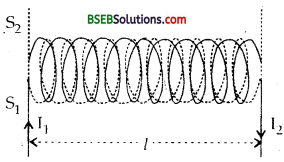Lei n1 and n2 be the no. of turns per unit length in S1 and S2 respectively.
If B1 be the magnetic field produced at any point inside S1 due to the current I1 in it, then
B1 = µ0n1I1 ……(1)
Now let ø2 be the magnetic flux linked with each turn of the solenoid.
ø2 = B1 x area of each turn = B1A …..(ii)
If ø be the total magnetic flux linked with S2, then
ø = ø2 x Total number of turns in S2.
= ø2 x n2x l = B1 A x n2l
= ø2n1l1An2l = µ0n1n2I1lA …….(iii)
Also ø ∝ F1 or ø = MI1 …….(iv)
∴ From (iii) and (iv), we get
MI1 = µ0n1n2lAI1
or M = µ0n1n2lA ……..(v)
If N1 and N2be the total no. of turns in each coil, then N1 = n1l and N2 = n2l.
∴ (v) reduces to
M = µ0 $$\frac{N_{1}}{l} \cdot \frac{N_{2}}{l}$$ Al
M = µ0 $$\frac{\mathbf{N}_{1} \mathbf{N}_{2} \mathbf{A}}{1}$$

Question 16.
What will happen if there is a relative motion between the magnet and the open coil?
In case of a coil having open ends, the induced e.m.f. is set up across the open ends of the coil due to flow of induced current when there is a relative motion between the magnet and the open coil.Question 17.
Prove that induced current in a coil is given by I ≈ $$\frac {N}{R}$$ A (B1 – B1) where the symbols have their usual mean.
We know from Q. 11 that
q = – $$\frac {1}{R}$$(ø2 – ø1)
Or L.dt = $$\frac {1}{R}$$ dø …….(1)
Where q = I dt = induced charged produced in the coil in a fine dt when a current I flows through it.
dø = ø2 – ø1 = change in magnetic flux.
∴ I = – $$\frac {1}{R}$$ $$\frac {dø}{dt}$$ ……(2)
When the magnetic field is parallel to the outward drawn normal to the surface of the coil, then θ = 0°.
∴ ø1 = B1 A and ø2 = B2A.
dø = (B2 – B1)A. ……(3)
If N be the total no. of turns in the coil, then ‘
dø = N (B2 – B1) A ……(4)
∴ From (2) and (4), we get
I = – $$\frac {1}{R}$$ N(B2 – B1) A
I = $$\frac {NA}{R}$$ (B2 – B1)

Question 18.
Explain why the word generator is a misnomer? Define A.C. generator.
A.C. generator simply converts mechanical energy into electrical energy. Thus it would be better to call it a converter. It is a device which converts stored chemical energy into alternating electrical energy.

Question 19.
Explain the working of A.C. generator.
As per Q1 (long answer type Question), the e.mi. induced by rotation of a coil in a magnetic field is given by
ε = NBA ω sin ωt = ε0,sin ωt
where ε0 = NBAco is the amplitude of e.m.f. produced in the rotating coil.
If Rbe the resistance of the circuit, then the instantaneous current produced in the circuit is given by :when I0 = $$\frac{\varepsilon_{0}}{\mathrm{R}}$$ is the maximum value of instantaneous current.

Question 1.
Explain a method of producing induced e.m.f. in a coil by changing its relative orientation in a magnetic field.
Consider a coil abed having N turns capable of rotating around its vertical axis YY’ in a horizontal magnetic field $$\overrightarrow{\mathrm{B}}$$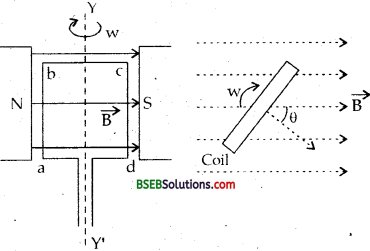When the plane of coil is parallel to $$\overrightarrow{\mathrm{B}}$$ , the magnetic flux linked with the coil is zero but when the plane of the coil is ⊥ar to the $$\overrightarrow{\mathrm{B}}$$ , the magnetic flux linked with the coil is maximum. Thus when the coil rotates along Y’Y axis in a magnetic field, the magnetic flux linked with the coil changes continuously from 0 to maximum and then from maximum to zero.
We know that when the magnetic flux through any closed circuit changes, induced e.m.f. produced in the circuit causes an increase in the magnetic flux and the induced current is produced in one direction. If the magnetic flux decreases, then the induced current decreases and is produced in a direction opposite to the previous one. Thus in this case induced current with continuously changing direction is obtained due to continuous rotation of the coil in the m agnetic field.

Magnitude of induced e.m.f. and current :
Let the coil be initially parallel to $$\overrightarrow{\mathrm{B}}$$ at t = 0, .
∴ θ = 90° where θ is the angle between outward normal to the surface of the coil and the magnetic field $$\overrightarrow{\mathrm{B}}$$
Let N be the no. of turns in the coil.
if ø be the magnetic flux linked with the coil after a time. Then
ø = NAB cos θ
where θ = ωt, ω = angular displacement.
ø = NBA cos ωt. ……….(1)
If e be the induced e.m.f. produced in the coil, then
e = – $$\frac {dø}{dt}$$ = – $$\frac {d}{dt}$$(ø) = – $$\frac {d}{dt}$$(NBA cos ωt)
= – NBA $$\frac {d}{dt}$$ cos ωt = NBA ω sin ωt ………..(2)
equation (2) shows that e varies sinusoidally with time.Numerical Problems

Question 1.
A wire 40 cm long, bent into rectangular loop 15 cm x 5 cm is placed perpendicular to the magnetic field of 0.08 wb m-2. After 0.5 second, the loop is changed into 10 cm square rod and flux density increases to 1.4 wbm-2. Calculate the value of induced e.m.f.
Here, Intial Area of loop, Al = 15 x 5 cm-2 = 75 x 10-4 m-2.
B1 = initial flux density = 0.08 Wb m-2
Final area of the loop, A2 = 10 x 10 cm2 = 100 x 104 m2.
B2 = final flux density = 1.4 Wbm-2.
If ø1 and ø2 be the initial and final magnetic flux linked with the loop, then
ø1 = B1A1 = 0.08 x 75 x 10-4 wb = 60 x 10-4 wb.
and ø2 = B2A2 = 1.4 x 100 x 10-4 Wb = 140 x 10-4wb.
∴ Change in magnetic flux, dø = ø2 – ø1 = 140 x 10-4 – 60 x 10-4 = 80 x 10-4 wb.
dt = time in which the magnetic flux takes place = 0.5 s.
e = induced e.m.f. = ?
e = – $$\frac {dø}{dt}$$ = – $$\frac{80 \times 10^{-4}}{0.5}$$ = 0.016 V.

Question 2.
The magnetic flux through a coil along its normal is given by the relation, = (5t-3 + 4t2 + 2t – 5) wb. Its resistance is 50 Q. Calculate the induced current through the coil at t = 2s.
Here, ø = 5t-3 + 4t2 + 2t – 5
R = 50 Ω
t = 2s
Let e = induced e.m.f
and I = magnitude of induced current m tne coil = ?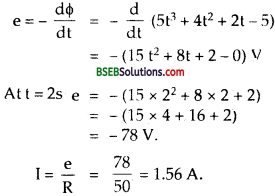Question 3.
A circular coil of diameter. 1 m is moved in a uniform magnetic field and an induced e.m.f. of 20 V is set up in 5 s. Find the magnetic flux and the magnetic field.
Here D = diameter of coil = 1 m.
∴ r = radius of coil = $$\frac {D}{2}$$ = 0.5 m = $$\frac {1}{2}$$ m
e = induced e.m.f. = -20 V.
dt = time = 5 s, B = ?, ø or dø = ?
If A be the area of the coil, then
A = π r2 = $$\frac {22}{7}$$ x ($$\frac {1}{2}$$)2 = $$\frac {11}{14}$$ m2
If dø be the change in magnetic flux, then
e = – $$\frac {dø}{dt}$$ or dø = – e dt = – (- 20) x S = 100 wb
∴ dø = BA
or B = $$\frac{\mathrm{d} \phi}{\mathrm{A}}=\frac{100}{\left(\frac{11}{14}\right)}=\frac{1400}{11}$$ = 127.27 TQuestion 4.
A closely wound rectangular coil of 200 turns and size 30 x 5 cm2 is placed perpendicular to the magnetic field of 0.20 T. Calculate induced e.m.f. it the coil when the magnetic field drops to 0.15 T in 0.02 s.
Here, A = area of coil = 30 x 5 cm2 = 150 x 10-4 m2
B1 = initial value of the magnetic field = 0.20 T
B2 = final value of the magnetic field = 0.15 T
dt = 0.02 s
N = no. of turns in the coil = 200.
e = induced e.m.f. in the coil = ?
Using the relation,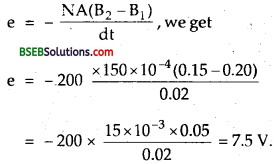Question 5.
A coil of 100 turns and area 0.1 m2 is perpendicular to a magnetic field of induction 4 x 10-3 wb m-2. If the coil is reversed in a 50 ms, calculate the induced e.m.f. in the coil.
Here, A = area of coil = 0.1 m2
N = no. of turns in the coil = 100
B = magnetic field = 4 x 10-3 wb m-2.
dt = time of change of magnetic flux = 50 ms = 50 x 10-3 s.
Let ø1 be the initial magnetic flux linked with the coil when it is ⊥ to $$\overrightarrow{\mathrm{B}}$$ i.e., θ1 = 0° between $$\overrightarrow{\mathrm{B}}$$ and $$\overrightarrow{\mathrm{A}}$$
ø1 = $$\overrightarrow{\mathrm{B}}$$ . $$\overrightarrow{\mathrm{A}}$$ = BA cos θ1= BA cos θ1 = BA
Also Let ø2 = final magnetic flux linked with the coil when coil is reversed i.e., θ2 = 180°.
ø2= BA cos 180° = BA (-1) = – BA
= – 4 x 10-3 x 0.1 = – 4 x 10-4 wb.
∴ dø = ø2 – ø1 = -4 x 10-4 – 4 x 10-4
= – 8 x 10-4 wb.
e = ?
∴ Using the relation
e = – N $$\frac {dø}{dt}$$ = – 100 x $$\frac{\left(-8 \times 10^{-4}\right)}{50 \times 10^{-3}}$$ = 1.6 VQuestion 6.
A conducting wire of 100 turns is wound over and near the centre of a solenoid of 100 cm length and 2 cm radius having 600 turns. Calculate the mutual inductance of the two coils.
Here, l = length of each solenoid = 100 cm = lm.
N1 = total no. of turns in first solenoid = 600
N2 = total no. of turns in 2nd solenoid = 100.
r = radius of first solenoid = 2 cm = 2 x 10-2 m.
∴ A = area of the solenoid = 7π-2 = n (2 x 10-2)2
= 4π x 10-4 m2.
M = mutual inductance of two coils = ?
Let n1 and n2 be the no. of turn per unit length of each solenoid,
n1 = $$\frac{N_{1}}{l}$$ = $$\frac {600}{1}$$ = 600 turns/m.
n2 = $$\frac{N_{2}}{l}$$ = $$\frac {600}{1}$$ = 600 turns/m.

∴ Using the relation,
M = μ0n1n2 Al, we get
M = 4π x 10-7 x 600 x 600 x 4π x 10-7 x l
= 96 x π2x 10-7H
= 96 x 9.87 x 10-7
= 9.47 x 10-5H.

Question 7.
A loop of wire is placed in a magnetic field $$\overrightarrow{\mathrm{B}}$$ = 0.5 $$\overrightarrow{\mathrm{B}}$$ T. If j the area of the loop is $$\overrightarrow{\mathrm{A}}$$ = ($$3 \hat{\mathbf{i}}+6 \hat{\mathbf{j}}+5 \hat{\mathbf{k}}$$) m2, then what is the angle between $$\overrightarrow{\mathrm{B}}$$ and $$\overrightarrow{\mathrm{A}}$$ ?
Here, $$\overrightarrow{\mathrm{B}}$$ = magnetic field = 0.5 $$\hat{\mathbf{i}}$$ T
A = area of loop = $$3 \hat{\mathbf{i}}+6 \hat{\mathbf{j}}+5 \hat{\mathbf{k}}$$ m2.
Let θ = angle between $$\overrightarrow{\mathrm{B}}$$ and $$\overrightarrow{\mathrm{A}}$$ = ?
We know that ø = $$\overrightarrow{\mathrm{B}}$$ , $$\overrightarrow{\mathrm{A}}$$ = BA cos θ.
cos θ = $$\frac{\vec{B} \cdot \vec{A}}{B A}$$ ……..(i)
Now $$\overrightarrow{\mathrm{B}}$$ . $$\overrightarrow{\mathrm{A}}$$ = $$\hat{\mathbf{i}}$$
= 0.5 x 3 $$\hat{\mathbf{i}}$$ . $$\hat{\mathbf{i}}$$ + 0.5 x 6 $$\hat{\mathbf{i}}$$ .$$\hat{\mathbf{j}}$$
+ 0.5 x 5 $$\hat{\mathbf{i}}$$ . $$\hat{\mathbf{k}}$$
= 1.5 x 1 + 3.0 x 0 + 2.5 x 0.
= 1.5 wb. …..(ii)
B = $$\sqrt{(1.5)^{2}+0^{2}+0^{2}}$$ = 0.5
A = $$\sqrt{3^{2}+6^{2}+5^{2}}$$
= $$\sqrt{9+36+25}=\sqrt{70}$$ ……..(iii)
∴ From (i), (ii) and (iii), we get
cos θ = $$\frac{1.5}{0.5 \times \sqrt{70}}=\frac{3}{\sqrt{70}}=\frac{3}{8.367}$$
= 0.3610
θ = 68°58′ = 68.98°.
≈ 69°.Question 8.
Find the minimum speed required to produce a potential difference of 5 V across the wings of an aeroplane 40 m in length due to the earth’s magnetic field (vertical component) of 5.0 x 105wbm-2.
Here, e = induced e.m.f. across the wings of the aeroplane = 5 V
Bv = vertical component of earth’s magnetic held = 5 x 10-5 wb m-2.
l = wing span = 40 m.
Let υ = minimum speed of the aeroplane = ?
Using the relation, e = Bvlυ, we get
υ = $$\frac{\mathrm{e}}{\mathrm{B}_{\mathrm{v}} l}=\frac{5}{5 \times 10^{-5} \times 40}=\frac{10^{5}}{40}$$
= 2500 ms-1.

Question 9.
A square metallic wire loop of side 10 cm and resistance 1Ω is moved with a constant velocity v-1 in a uniform magnetic field of induction 0.2 T as shown in the figure here. The magnetic field is perpendicular to the plane of the loop. The loop is connected to a network of resistance each of value 3Ω. The resistance of the lead wires QA and PB are negligible. What should be the speed of the loop so as to have a steady current of 1 mA in the loop?Here, $$\overrightarrow{\mathrm{B}}$$ = magnetic field = 2.0 T and it acts ⊥to the plane of paper away from us.
l = side of the square loop =10 m = 0.1 m
$$\overrightarrow{\mathrm{v}}$$ = velocity of loop ⊥ ar to $$\overrightarrow{\mathrm{B}}$$ =?
I = steady current in the loop = 1 mA = 1 x 10-3 A.
Value of each resistance of the network = 3 Ω.
The network is a balanced wheatstone bridge, so no current flows in arm CD.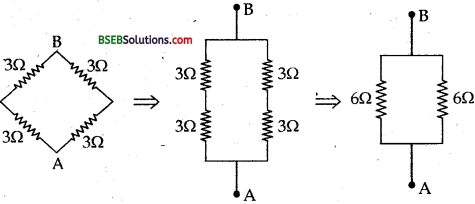Thus if R1 be the equivalent resistance of the network between the points A and B, then it reduces as
$$\frac{1}{R_{1}}=\frac{1}{6}+\frac{1}{6}$$ = $$\frac{2}{6}=\frac{1}{3}$$
∴ R1 = 3 Ω

Also R = resistance of loop = 1 Ω Now R and R1 are in series. Thus
if R’ be the equivalent resistance, then
R’ = R1 + R2 + R = 3 + 1 = 4 Ω .
if e be the induced e.m.f. produced in the ioop, then
e = Blυ
∴ I = $$\frac{e}{R}=\frac{B l v}{R}$$
or υ = $$\frac{I R}{B l}$$ = $$\frac{1 \times 10^{-3} \times 4}{2 \times 0.1}$$ = 20 x 10-3 ms-3 = 2 cm-1Question 10.
A small coil with an area 100 mm2 has 50 turns. The coil is placed between the pole pieces of a small magnet and then suddenly taken out. An induced e.m.f. of 0.05 V is induced in the coil in 50 ms when it is pulled to a field free region. What is the magnetic intensity between the poles? What was the original flux through each turn?
Here, A = area of the.core = 100 mm2 = 100 x 10-6 m2
N = total no. of turns in the coil =50
e = induced e.m.f. = 0.05 V
dt = time = 50 ms = 50 x 10-3s.
Let B = magnetic intensity = ?
ø = original magnetic flux with each turn = ?
Using the following relating the flux linked with the coil is given by :
ø1 = NBA
ø1 = 50 x B x 10-4
When the coil is taken out of the field,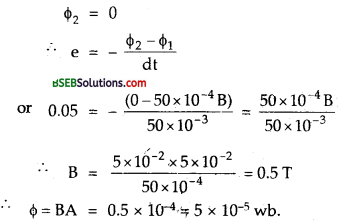Question 11.
A rectangular loop of sides 0.10 m and 0.03 m with a small cutis moving out of a region of uniform magnetic field of magnitude 0.5 T directed normal to the loop. What is the voltage developed across the cut if the velocity of the loop is 0.01 ms1 in a direction.
(i) normal to shorter side.
(ii) normalto long side.
(iii) For how long does the induced voltage last in each case ?
(iv) Is a force required to pull the loop if it has a cut ?
(v) Find the force needed to pull the loop normal to the shorter side if it has no cut and a resistance of 1 m?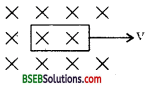Here, l = 0.10 m,b = 0.03 m, v = 0.01 ms-1, A = l x b = 3 x 10-3
m2, B = 0.5 T.
(i) e1 = Bυl= 0.5 x 10-2 x 3 x 10-2 = 1.5 x 10-14 V
(ii) e1 = 0.5 x 10-2 x 3 x 10-2= 1.5 x 10-4V
(iii) t1 = $$\frac{l}{υ}$$ = $$\frac{0.10}{1 \times 10^{-2}}$$ = 10 s.
∴ t2 = $$\frac{l}{υ}$$ = $$\frac{0.03}{1 \times 10^{-2}}$$ = 3 s
(iv) As there is no current in the loop, thus there is no 12R loss (i.e., power loss). Also there is no friction, hence no force is required to pull the loop. When it has a cut.
(v) Here, R = 1 m Ω = 10-3Question 12.
In a car spark coil, the e.m.f. of 4 x 104 V is induced in the secondary coil when the primary current changes from 4 A to 0A in 1C ps. Find the mutual inductance between the primary and the secondary windings of this spark coi1.
Here, e = induced e.m.f. in the coil = 4 x 104 V
initial current, I1 = 4 A
final current, I2 = 0A
dI = I2 – I1 = 0 – 4 = -4 A
dt = 10 µs = = 10 x 10-6s.
M = ?
Using the relation,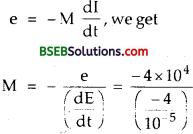= 0.1 H.

Question 13.
If self inductance of an air core solenoid increases from 0.04 mH to 16 mH on introducing an iron core into it, what is relative magnetic permeability of the core?
Here, L0 = Self inductance of air core solenoid = 0.04 mH = 4 x 10-5 H.
L = self inductance of iron core solenoid = 16 mH = 16 x 10-3 H.
µr = relative magnetic permeability of the core = ?
Let l = length of the solenoid
A = its area of cross-section
n = no. of turns per unit length of the solenoid.
∴ Using the relation, L = µn2 l A, we get
L0 = µ0n2lA ……..(1)
and L = µn2lA ……..(2)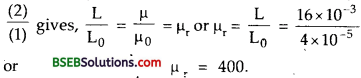Question 14.
A coil of wire of certain radius has 600 turns and a self inductance of 108 mH. What will be the self inductance of a similar coil having 500 turns.
Here, N1 = no. of turns in a coil = 600
N2= no. of turns in a second coil = 500
Let r and l be the radius and length of the coils.
A = Area of each col = πr2.
If L1and L2 be the self inductance of the two coils, then L1 = 108 mH – 108 x 10-3 H.
L2 = ?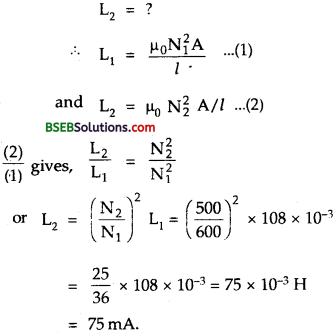Question 15.
A coil has a self inductance of 10 mH. What is the maximum magnitude of the induced e.m.f. in the inductor when a current I = 0.1 sin 2001A is sent through it.
Here, L = self inductance of the coil = 10 mH = 10 x 10-3 H.
I = 0.1 sin 200 tA
emax = induced e.m.f. in the coil = ?
We know that the induced e.m.f. in an inductor is given by e = L $$\frac{dl}{dt}$$
Fore to be max = emax, $$\frac{dl}{dt}$$ must be maximum i.e., = ($$\frac{dl}{dt}$$)max
emax = L ($$\frac{dl}{dt}$$)max
Now $$\frac{dl}{dt}$$ = (0.1 cos 200 t) x 200 = 20 cos 200 t
$$\frac{dl}{dt}$$ will be maximum, when cos 200 t = max. = 1 = cos 0.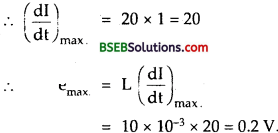Question 16.
A copper rod of length 0.5 m rotates about one of its ends at angular velocity of 32 rad s-1 in a uniform magnetic field 0.4 wb m-2 the plane of rotation being at right angles to the field. Find the e.m.f. developed between the ends of the rod.
Here, l = length of the rod = 0.5 m
ω = angular speed of the rod about one of its ends = 32 rad s-1.
B = uniform magnetic field = 0.4 wb m-2.
A = area swept in one complete rotation = 7πr2
= πl2 (∴ r = l here)
If t = time taken in one complete rotation, then
t = $$\frac{2π}{ω}$$
Let e = induced e.m..f. produced between the ends of the rod = ?
Let ø = BA be the total magnetic flux linked with each turn of the coil.Question 17.
A metallic wire bent in the form of a semi-circle of radius 0.1 m is moved in a direction parallel to its plane but normal to a magnetic field B = 20 mT with a velocity of 10 ms-1. Find the e.m.f. induced in the wire.
Here, υ = velocity = 10 ms-1.
B = magnetic field = 20 mT = 20 x 10-3 T.
r = radius of semi-circular wire = 0.1 m.
e = induced e.m.f. = ?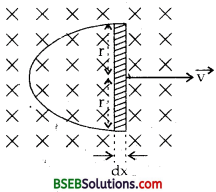Let dx = distance moved by the semi-circular wire in or out of $$\overrightarrow{\mathrm{B}}$$ in a time dt.
∴ A = area swept in time dt
= 2r x dx
∴If ø be the magnet- flux linked with area A, then
ø = BA = B. 2r dx (∴ $$\overrightarrow{\mathrm{A}}$$ is ⊥ to $$\overrightarrow{\mathrm{B}}$$ )
e = $$\frac{dø }{dt}$$ = $$\frac{d}{dt}$$ (o) = $$\frac{d}{dt}$$ (B. 2r dx)
= 2r B $$\frac{dx}{dt}$$ = 2r B υ
= 2 x 0.1 x 20 x 10-3 x 10
= 40 x 10-3 V
= 0.04 V.Question 18.
A horizontal copper disc of radius 10 cm rotates about a vertical axis passing through its centre with a frequency of 10 revolutions per second. A uniform magnetic field of 100 G acts perpendicular to the plane of disc. Calculate the potential difference between the centre and rim.
Here, r = radius of copper disc = 10 cm = 0.10 m.
v = frequency of revolution = 10 cps.
B = 100 G = 100 x 10-4 T = 10-2T.
θ = 90° between $$\overrightarrow{\mathrm{A}}$$ and $$\overrightarrow{\mathrm{B}}$$
Let A = area of disc
∴ ø = magnetic flux linked with the disc
= $$\overrightarrow{\mathrm{B}}$$ . $$\overrightarrow{\mathrm{A}}$$ = BA cos 0 = BA.
$$\frac{dA}{dt}$$ = Area swept out by the disc per sec.= A. v = πr2v.
If e be the potential difference or induced e.m.f. between the centre of the disc and the trim, thenQuestion 19.
A long solenoid of diameter 0.1 m has 2 x 104 turns per metre. At the centre of the solenoid a 100 turn coil of radius 0.01 m is placed with its axis coinciding with the axis of solenoid. The current in the solenoid is decreased at a constant rate from + 2A to – 2A in 0.05 s. Find the e.m.f. induced in the coil. Also find the total charge flowing through the coil during this time when the resistance of the coil is 107t2 ohms.
Here, n = no. of turns per unit length of the solenoid = 2 x 104 tums/m.
l = length of the solenoid = 0.1 m
Let l1 = initial current flowing through the solenoid. = + 2A.
I2 = final current in the solenoid = – 2 A.
∴ dl = change in current = I2 – I1, = -2 – 2 = – 4A
dt = time in which change in current takes place = 0.05 s.
∴ $$\frac{dl}{dt}$$ = $$\frac{-4}{0.05}$$ As-1 = – 80 As-1
N = total no. of turns in the coil = 100
r = radius of the coil placed at the centre of the solenoid = 0.01 m.
e = induced e.m.f. in the coil = ?
q = total charge flowing through the coil = ?
R = resistance of coil = 10πr2
If B be the magnetic field produced at the centre of the solenoid, then
B = μ0nI and it acts along the axis of the solenoid and axis of the coil (placed inside the solenoid) coincides with the axis, of the solenoid,
hence the plane of the coil is ⊥ ar to $$\overrightarrow{\mathrm{B}}$$ .
∴If ø be the flux linked with each turn of the coil, then
ø = BA = μ0nIA. wb.
where A = πr2 for coil
= π x (0.01)2
∴ According to Faraday’s law of e.m.f., induced e.m.f. produced in a coil of N turns is given byFill In The Blanks

Question 1.
If a core of soft iron is introduced into a coil its coefficient of self induction gets ………..
increased.

Question 2.
Two circular conductors A and B are placed perpendicular to each other as shown in the figure. If the current in one of the coils is changed, then current induced in the other coil is equal to …………
Zero.Question 3.
The coils in the resistance boxes are made from ……….
double wire.

Question 4.
When the rate of change of current through a closed circuit is unity, then the induced e.m.f. produced in it is equal to ……….
Coefficient of self induction or self inductance L.

Question 5.
A copper rod of length l is rotated about one end perpendicular to the uniforfh field B with constant angular velocity to. The induced e.m.f. between its two end is …………
$$\frac {1}{2}$$ B ωl2Question 6.
If a coil is removed from a magnetic field : (a) slowly, (b) rapidly, then work done will be more when the coil is removed from the magnetic field ………..
rapidly.

Question 7.
A coil having area A, total number of turns N is rotated in a uniform magnetic field $$\overrightarrow{\mathrm{B}}$$ with an angular velocity ca. The maximum e.m.f. induced in the coil is equal to …………..
NBAco.

Question 8.
A coil of copper wire is being pulled with a constant velocity $$\overrightarrow{\mathrm{υ}}$$ in a magnetic field $$\overrightarrow{\mathrm{B}}$$ . If its ohmic resistance is increased, it will be ……….. to pull it.
easier.

Question 9.
If in Q. 8 above, the magnetic field be doubled, then the force required to pull the coil will become …………. the initial value.
four times (Hint: F ∝B2).

Question 10.
A coil of resistance R and inductance L is connected to a battery of e.m.f. E volts. The final current in the coil is ………..
$$\frac{E}{R}$$

Question 11.
A 50 mH coil carries a current of 2A. The energy stored in it is ……….. J.
0.1 (Hint: E = $$\frac{1}{2}$$LI2).

Question 12.
A device that rely upon the relationship between the electric current and the magnetic field is ……….. and ………..
Galvanometer, Generator, electric motor.

Question 13.
When the number of turns in a coil is doubled without any change in the length of the coil, its self inductance becomes …………
four times (Hint: L ∝ N2).

Question 14.
The induced e.m.f. produced in a wire of length / moving in a magnetic field $$\overrightarrow{\mathrm{B}}$$ with a constant velocity v is given by ……….. the induced current in a loop of this wire having resistance R is ………..
B υ l, $$\frac{Bυl}{R}$$Question 15.
The normal drawn to the surface of a conductor makes an angle θ with the direction of magnetic field $$\overrightarrow{\mathrm{B}}$$ The magnetic flux ø passing through the area A is …………..
ø = $$\overrightarrow{\mathrm{B}}$$ . $$\overrightarrow{\mathrm{A}}$$

Question 16.
The magnetic energy stored in a solenoid in terms of B, A and length of solenoid is …………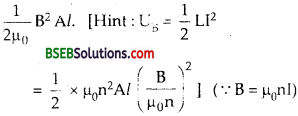$$\frac{\mathrm{B}^{2}}{2 \mu_{0}}$$
A circular coil of N turns and radius r is placed in a uniform magnetic field $$\overrightarrow{\mathrm{B}}$$. Initially the plane of coil is perpendicular to the magnetic field. The coil is then rotated by 90°. If the resistance of the oil is R, then the charge passing through the coil is given by ………….
$$\frac{n B \pi r^{2}}{R}$$